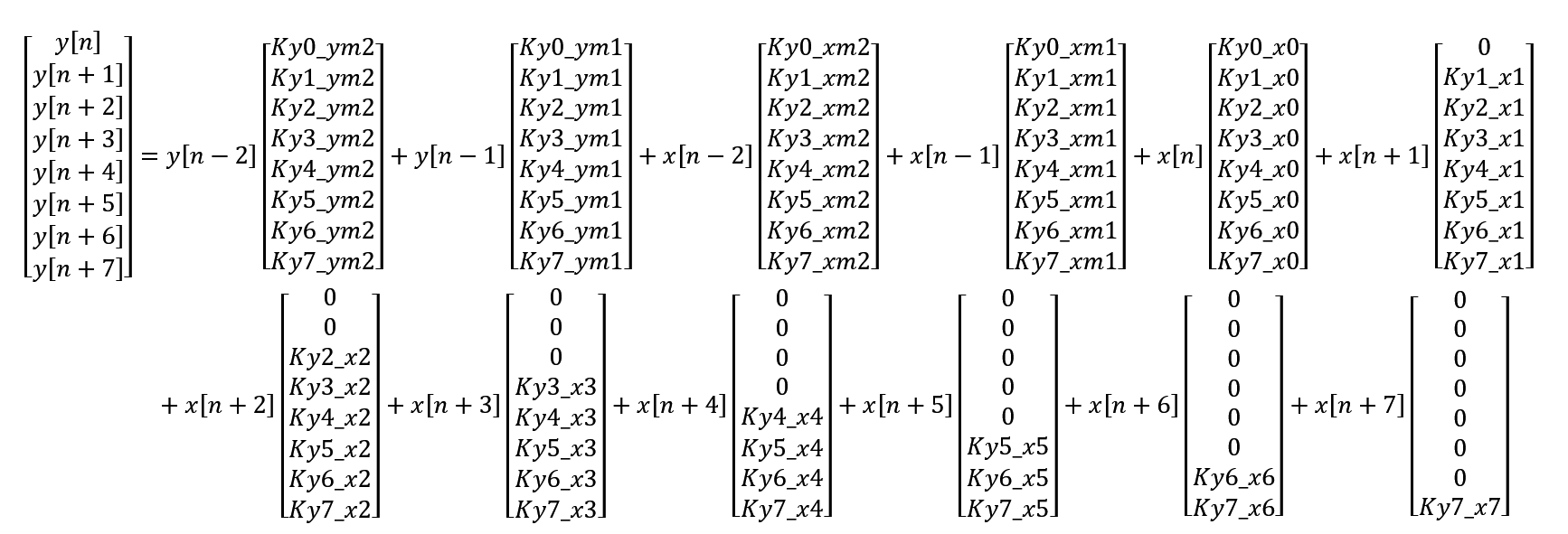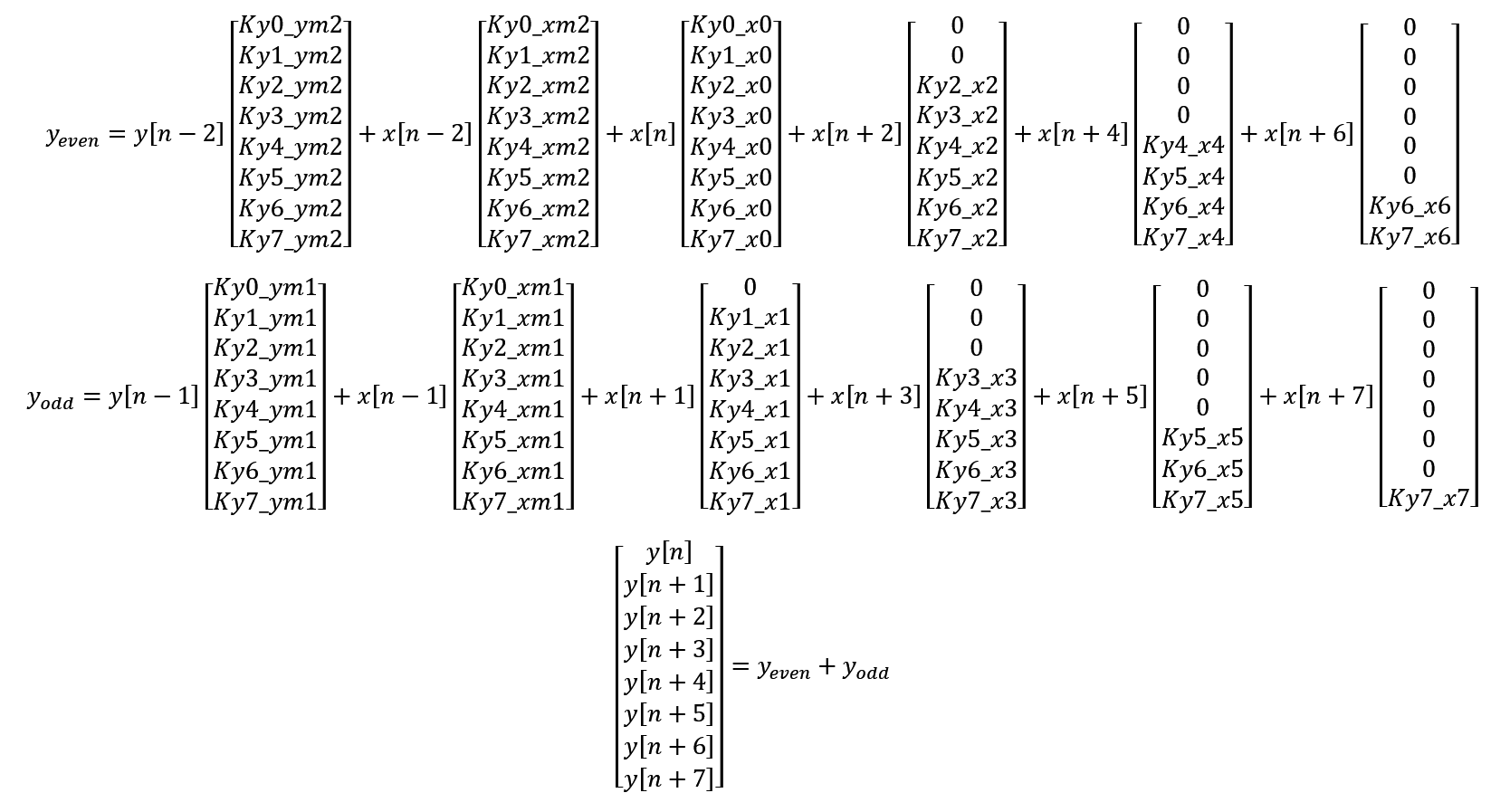# Preliminaries - 2022.2 English

## Vitis Tutorials: AI Engine Development

Document ID
XD100
Release Date
2022-12-01
Version
2022.2 English

In Part 2a, we examined the generated assembler code and found that there is a `NOP` (no operation) between the `VFPMAC` (vector floating-point multiply-accumulate) mnemonics. This `NOP` is unavoidable as a floating-point accumulation requires 2 cycles (see Fig. 26 of AM009).

There are 2 possible solutions to “squeeze out” the `NOPs` to allow a floating-point multiply-accumulate on each cycle.

• split the matrix-vector multiplication into 2 separate multiply-accumulate operations such that a floating-point accumulation can be performed on each cycle

• use fixed-point (which uses one cycle for accumulation)

We will focus on splitting the floating-point matrix-vector multiplication in this section.

Note that instead of the “traditional” method of multiplying each row of the matrix by the column vector, we are effectively scaling each column of the matrix by the corresponding element in the vector with the multiply-accumulate API.Thus, splitting the vector additions into even and odd parts will allow us to perform independent multiply-accumulate operations:Also note that the AI engine has 2 load units. The Julia program `aie_iir_2b.jl` has been modified to split the matrix into even and odd columns and generate two separate header files.

We start by using the AI Engine APIs.